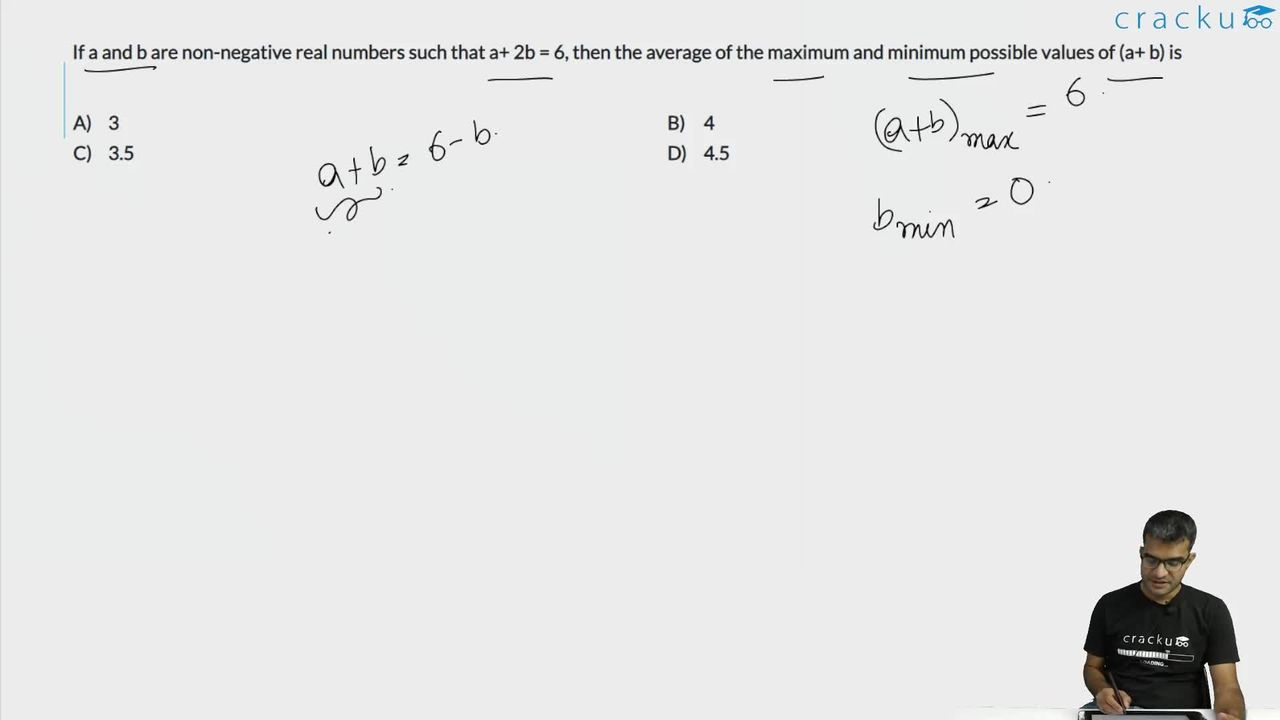Question 20

# If a and b are non-negative real numbers such that a+ 2b = 6, then the average of the maximum and minimum possible values of (a+ b) is

Solution

a + 2b = 6

From the above equation, we can say that maximum value b can take is 3 and minimum value b can take is 0.

a + b + b = 6

a + b = 6 - b

a + b is maximum when b is minimum, i.e. b = 0

Maximum value of a + b = 6 - 0 = 6

a + b is minimum when b is maximum, i.e. b = 3

Minimum value of a + b = 6 - 3 = 3

Average = $$\ \frac{\ 6+3}{2}$$ = 4.5

### View Video Solution